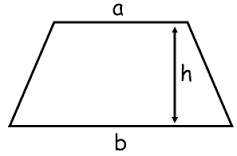## Trapezium Calculator

Calculate the various properties of Trapezium for given values. A Trapezium is a quadrilateral with one pair of parallel sides.
Both UK and US definitions of trapezoid and trapezium are swapped over. The trapezium is called a trapezoid in the UK.Area of Trapezium/Trapezoid:[ ½×(sum of parallel sides)×(distance between them) ] Enter the size of parallel side 1 = Enter the size of parallel side 2 = Enter the distance between parallel sides = Area of Trapezium =Perimeter of Trapezium/Trapezoid:[ (sum of all four sides) ] Enter the length of the sides = Perimeter of Trapezium =